# Deep Learning Performance 1 Batch Size, Epochs and Optimizers¶

## Batch Size and Epochs¶

### What Is a Batch?¶Neural networks are trained using gradient descent where the estimate of the error is used to update the weights and is calculated based on a subset of the training dataset. The number of examples from the training dataset used in the estimate of the error gradient is called the batch size and is an important hyperparameter that influences the dynamics of the learning algorithm.

• Batch size controls the accuracy of the estimate of the error gradient when training neural networks.
• There is a tension between batch size and the speed and stability of the learning process.
• Smaller batch sized can work their way out of "local minima" more easily.
• Batch sizes that are to large can get end up getting stuck in the wrong solution.

#### Flavors of Batch Gradient Descent:¶

• Batch Gradient Descent: Batch Size = Size of Training Set
• Stochastic Gradient Descent: Batch Size = $1$
• Mini-Batch Gradient Descent: Batch size is set to more than one and less than the total number of examples in the training dataset

In the case of mini-batch gradient descent, popular batch sizes include $32$, $64$, and $128$ samples. You may see these values used in models often in deep learning literature.

• The frequent updates immediately give an insight into the performance of the model and the rate of improvement.
• The increased model update frequency can result in faster learning on some problems.
• The noisy update process can allow the model to avoid local minima (e.g. premature convergence).

Downsides:

• Updating the model so frequently is more computationally expensive than other configurations of gradient descent, taking significantly longer to train models on large datasets.
• The frequent updates can result in a noisy gradient signal, which may cause the model parameters and in turn the model error to jump around (have a higher variance over training epochs).
• The noisy learning process down the error gradient can also make it hard for the algorithm to settle on an error minimum for the model.

• Fewer updates to the model means this variant of gradient descent is more computationally efficient than stochastic gradient descent.
• The decreased update frequency results in a more stable error gradient and may result in a more stable convergence on some problems.
• The separation of the calculation of prediction errors and the model update lends the algorithm to parallel processing based implementations.

• The more stable error gradient may result in premature convergence of the model to a less optimal set of parameters.
• The updates at the end of the training epoch require the additional complexity of accumulating prediction errors across all training examples.
• Commonly, batch gradient descent is implemented in such a way that it requires the entire training dataset in memory and available to the algorithm.
• Model updates, and in turn training speed, may become very slow for large datasets.

• The model update frequency is higher than batch gradient descent which allows for a more robust convergence, avoiding local minima.
• The batched updates provide a computationally more efficient process than stochastic gradient descent.
• The batching allows both the efficiency of not having all training data in memory and algorithm implementations.

• Mini-batch requires the configuration of an additional “mini-batch size” hyperparameter for the learning algorithm.
• Error information must be accumulated across mini-batches of training examples like batch gradient descent.

## Optimizing Batch Size Multi-Class Classification Problem¶

We will use a small multi-class classification problem as the basis to demonstrate the effect of batch size on learning.

The scikit-learn class provides the make_blobs() function that can be used to create a multi-class classification problem with the prescribed number of samples, input variables, classes, and variance of samples within a class.

The problem can be configured to have two input variables (to represent the $x$ and $y$ coordinates of the points) and a standard deviation of $2.0$ for points within each group. We will use the same random state (seed for the pseudorandom number generator) to ensure that we always get the same data points.

In :
# scatter plot of blobs dataset
from sklearn.datasets import make_blobs
import matplotlib.pyplot as plt
import numpy as np

# generate 2d classification dataset
X, y = make_blobs(n_samples=1000, centers=3, n_features=2, cluster_std=2, random_state=2)

# scatter plot for each class value
for class_value in range(3):

# select indices of points with the class label
row_ix = np.where(y == class_value)

# scatter plot for points with a different color
plt.scatter(X[row_ix, 0], X[row_ix, 1], label=class_value, alpha=0.5)
plt.legend()

# show plot
plt.show()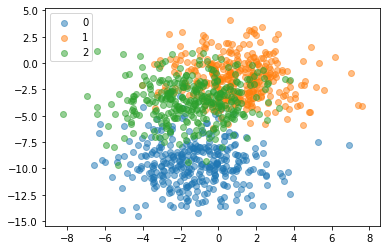We can see that the standard deviation of $2.0$ means that the classes are not linearly separable (separable by a line) causing many ambiguous points.

This is desirable as it means that the problem is non-trivial and will allow a neural network model to find many different “good enough” candidate solutions.

In :
from keras.layers import Dense
from keras.models import Sequential
from keras.optimizers import SGD
from keras.utils import to_categorical

# prepare train and test dataset
def prepare_data():

# generate 2d classification dataset
X, y = make_blobs(n_samples=1000, centers=3, n_features=2, cluster_std=2, random_state=2)

# one hot encode output variable
y = to_categorical(y)

# split into train and test
n_train = 500
train_X, test_X = X[:n_train, :], X[n_train:, :]
train_y, test_y = y[:n_train], y[n_train:]
return train_X, train_y, test_X, test_y

# fit a model and plot learning curve
def fit_model(train_X, train_y, test_X, test_y, n_batch, epochs, opt):

# define model
model = Sequential()

# compile model
model.compile(loss='categorical_crossentropy', optimizer=opt, metrics=['accuracy'])

# fit model
history = model.fit(train_X, train_y, validation_data=(test_X, test_y), epochs=epochs, verbose=0,
batch_size=n_batch)

# evaluate the model
scores = model.evaluate(test_X, test_y)
accuracy = round(scores * 100, 3)

# plot learning curves
plt.plot(history.history['accuracy'], label='train')
plt.plot(history.history['val_accuracy'], label='test')
plt.title(f'Accuracy = {accuracy}%, Batch Size = {n_batch}' + \
f', \n# of Epochs = {epochs}', pad= -40)

plt.legend()

Using TensorFlow backend.

In :
# prepare dataset
train_X, train_y, test_X, test_y = prepare_data()

# create learning curves for different batch sizes
batch_sizes = [5, 10, 16, 32, 64, 128, 256, len(X)]

# set optimizer
opt = SGD(lr=0.01, momentum=0.9)

plt.figure(figsize=(12,12))

for i in range(len(batch_sizes)):

# determine the plot number
plot_no = 420 + (i+1)
plt.subplot(plot_no)

# fit model and plot learning curves
fit_model(train_X, train_y, test_X, test_y, batch_sizes[i], epochs=200, opt=opt)

# show learning curves
plt.tight_layout()
plt.show()

500/500 [==============================] - 0s 11us/step
500/500 [==============================] - 0s 10us/step
500/500 [==============================] - 0s 10us/step
500/500 [==============================] - 0s 13us/step
500/500 [==============================] - 0s 11us/step
500/500 [==============================] - 0s 13us/step
500/500 [==============================] - 0s 10us/step
500/500 [==============================] - 0s 10us/step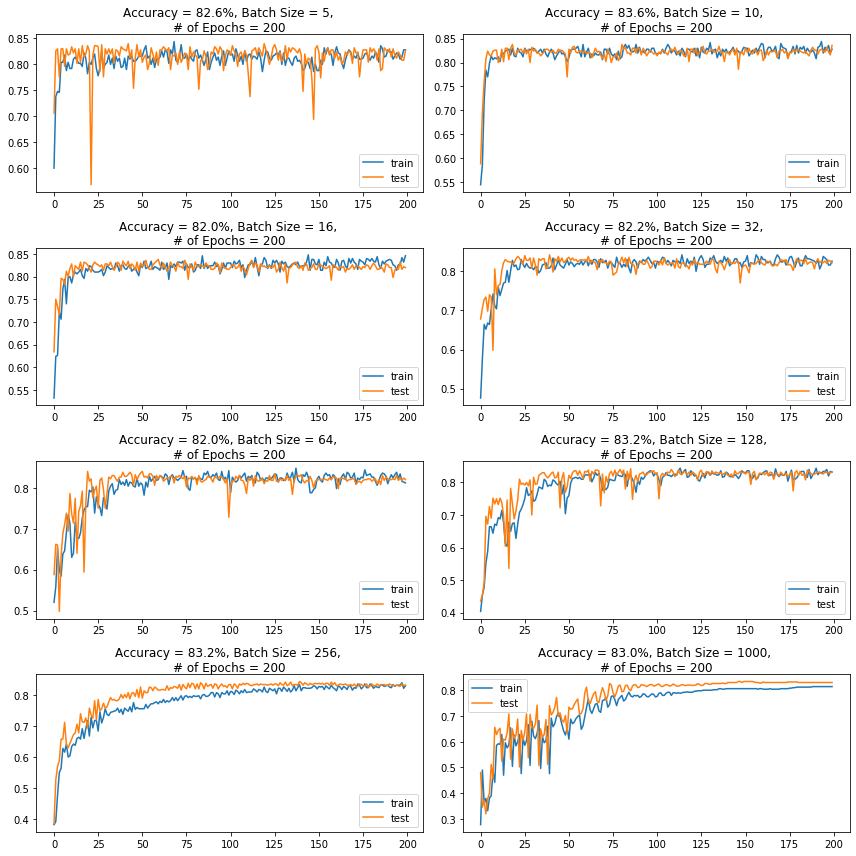Running the example creates a figure with eight line plots showing the classification accuracy on the train and test sets of models with different batch sizes when using mini-batch gradient descent.

The plots show that small batch results generally in rapid learning but a volatile learning process with higher variance in the classification accuracy. Larger batch sizes slow down the learning process but the final stages result in a convergence to a more stable model exemplified by lower variance in classification accuracy.

## What Is an Epoch?¶

The number of epochs is a hyperparameter that defines the number times that the learning algorithm will work through the entire training dataset.

$1$ epoch means that each sample in the training dataset has had an opportunity to update the internal model parameters. An epoch is comprised of one or more batches. For example, as above, an epoch that has one batch is called batch gradient descent.

You can think of it as a for-loop over the number of epochs where each loop proceeds over the training dataset. Within this for-loop is another nested for-loop that iterates over each batch of samples, where $1$ batch has the specified “batch size” number of samples to estimate error and update weights.

The number of epochs is traditionally large, often hundreds or thousands, allowing the learning algorithm to run until the error from the model has been sufficiently minimized. You may see examples of the number of epochs in the literature and in tutorials set to $10$, $100$, $500$, $1000$, or even larger.

### Optimizing Number of Epochs Multi-Class Classification Problem¶

We will use the same dataset we used to look at batch size to look at the effect of the number of epochs on the models learning.

In :
# create learning curves for different epoch sizes
n_epochs = [10, 20, 32, 64, 128, 200, 400, 500]

# set optimizer
opt = SGD(lr=0.01, momentum=0.9)

plt.figure(figsize=(12,12))

for i in range(len(n_epochs)):

# determine the plot number
plot_no = 420 + (i+1)
plt.subplot(plot_no)

# fit model and plot learning curves
fit_model(train_X, train_y, test_X, test_y, 256, epochs=n_epochs[i], opt=opt)

# show learning curves
plt.tight_layout()
plt.show()

500/500 [==============================] - 0s 10us/step
500/500 [==============================] - 0s 12us/step
500/500 [==============================] - 0s 12us/step
500/500 [==============================] - 0s 10us/step
500/500 [==============================] - 0s 12us/step
500/500 [==============================] - 0s 10us/step
500/500 [==============================] - 0s 11us/step
500/500 [==============================] - 0s 11us/step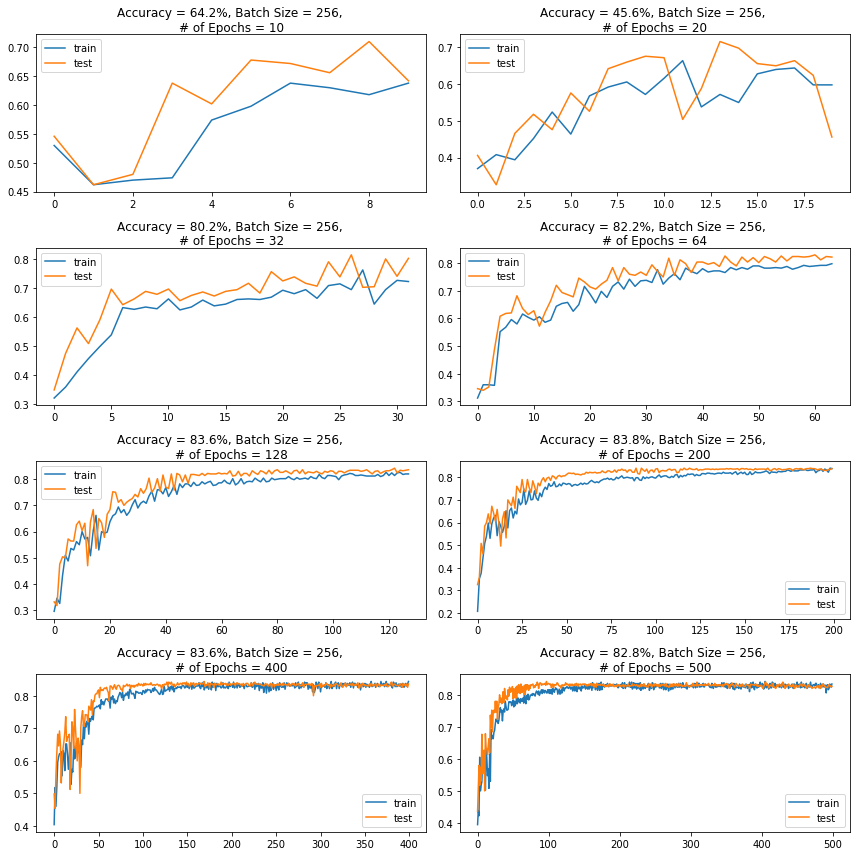Running the example creates a figure with eight line plots showing the classification accuracy on the train and test sets of models with a different number of epochs.

The plots show that a small number of epochs results in a volatile learning process with higher variance in the classification accuracy. A larger number of epochs result in a convergence to a more stable model exemplified by lower variance in classification accuracy.

## What are Optimizers?¶

Optimizers are algorithms or methods used to change the attributes of your neural network such as weights and learning rate in order to reduce the losses.

How you should change your weights or learning rates of your neural network to reduce the losses is defined by the optimizers you use. Optimization algorithms or strategies are responsible for reducing the losses and to provide the most accurate results possible.

Available TensorFlow Optimizers (weight update rule):

• SGD
• RMSprop

It’s a variant of Gradient Descent. It tries to update the model’s parameters more frequently. In this, the model parameters are altered after computation of loss on each training example.

Stochastic gradient descent (SGD) performs a parameter update for each training example $x^{(i)}$ and label $y^{(i)}$

$$\theta = \theta - \eta \cdot \nabla_\theta J( \theta; x^{(i)}; y^{(i)})$$

As the model parameters are frequently updated parameters have high variance and fluctuations in loss functions at different intensities.

• Frequent updates of model parameters hence, converges in less time.
• Requires less memory as no need to store values of loss functions.
• May get new minima’s.

• High variance in model parameters.
• May overshoot even after achieving global minima.
• To get the same convergence as gradient descent it needs to slowly reduce the value of learning rate.

One of the disadvantages of all the optimizers explained is that the learning rate is constant for all parameters and for each cycle. This optimizer changes the learning rate. It changes the learning rate $\eta$ for each parameter and at every time step $t$. It’s a type second order optimization algorithm. It works on the derivative of an error function.

$$g_{t, i} = \nabla_\theta J( \theta_{t, i} )$$

A derivative of loss function ($\nabla$, nabla) for every weight ($\theta$, theata) in the neural network with respect to the error function ($J$) at a given time $t$ and input $i$

$$\theta_{t+1, i} = \theta_{t, i} - \dfrac{\eta}{\sqrt{G_{t, ii} + \epsilon}} \cdot g_{t, i}$$

Update weights ($\theta$, theata) for given input $i$ and at time/iteration $t$

$\eta$ is a learning rate which is modified for given parameter $\theta_{i}$ at a given time based on previous gradients calculated for given parameter $\theta_{i}$.

We store the sum of the squares of the gradients with respect to $\theta_{i}$ up to time step t, while $\epsilon$ is a smoothing term that avoids division by zero (usually on the order of $1e^{−8}$. Interestingly, without the square root operation, the algorithm performs much worse.

It makes big updates for less frequent parameters and a small step for frequent parameters.

• Learning rate changes for each training parameter.
• Don’t need to manually tune the learning rate.
• Able to train on sparse data.

• Computationally expensive as a need to calculate the second order derivative.
• The learning rate is always decreasing results in slow training.

It is an extension of AdaGrad which tends to remove the decaying learning rate problem of it. Instead of accumulating all previously squared gradients, Adadelta limits the window of accumulated past gradients to some fixed size $w$. In this exponentially moving average is used rather than the sum of all the gradients.

$$E[g^2]_t = \gamma E[g^2]_{t-1} + (1 - \gamma) g^2_t$$

We set $\gamma$ to a similar value as the momentum term, around $0.9$. For clarity, we now rewrite our vanilla SGD update in terms of the parameter update vector $\Delta \theta_{t}$

$$\Delta \theta_t = - \dfrac{\eta}{\sqrt{E[g^2]_t + \epsilon}} g_{t}$$

Update the parameters

• Now the learning rate does not decay and the training does not stop.

• Computationally expensive.

Adam (Adaptive Moment Estimation) works with momentums of first and second order. The intuition behind the Adam is that we don’t want to roll so fast just because we can jump over the minimum, we want to decrease the velocity a little bit for a careful search. In addition to storing an exponentially decaying average of past squared gradients like AdaDelta, Adam also keeps an exponentially decaying average of past gradients $M_t$.

$M_t$ and $V_t$ are values of the first moment which is the Mean and the second moment which is the uncentered variance of the gradients respectively.

\begin{align} \begin{split} \hat{m}_t &= \dfrac{m_t}{1 - \beta^t_1} \\ \hat{v}_t &= \dfrac{v_t}{1 - \beta^t_2} \end{split} \end{align}

First and second order of momentum

To update the parameter:

$$\theta_{t+1} = \theta_{t} - \dfrac{\eta}{\sqrt{\hat{v}_t} + \epsilon} \hat{m}_t$$

The default values are $0.9$ for $\beta_1$, $0.999$ for $\beta_2$, and $10^{-8}$ for $\epsilon$

• The method is too fast and converges rapidly.
• Rectifies vanishing learning rate, high variance.

• Computationally expensive.

Comparison between various optimizers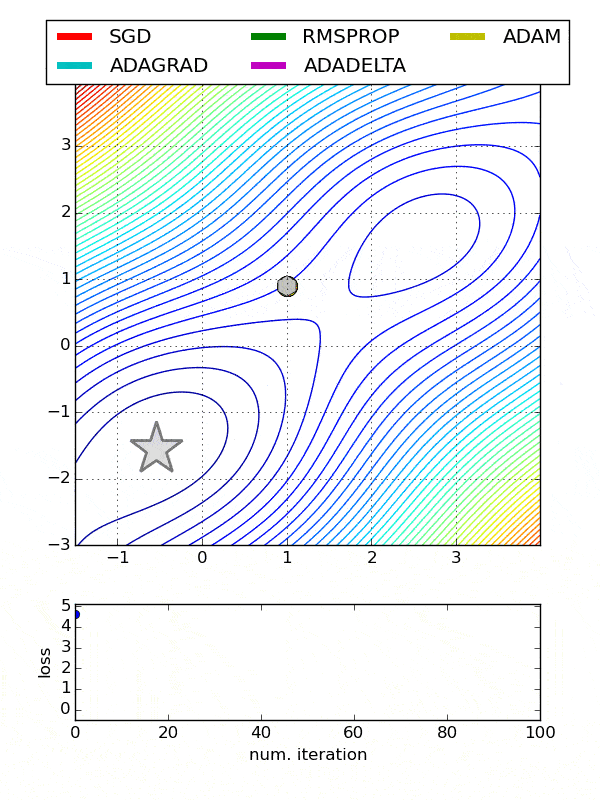### Choosing an Optimizer Multi-Class Classification Problem¶

We will use the same dataset we used previously to look at the choice of optimizer and the effects on the models learning.

In :
from keras.optimizers import Adam, Adamax, Adagrad, Adadelta, RMSprop

# set optimizer and learning rates for each
opt0 = SGD(lr=0.01, momentum=0.9)
opt5 = RMSprop(lr=0.01)

# create dictionary of optimizers to iterate through
opt_dict = {'SGD':      opt0,
'RMSprop':  opt5,
}

In :
# fit a model and plot learning curve
def fit_model_opt(train_X, train_y, test_X, test_y, n_batch, epochs, opt, opt_name='SGD'):

# define model
model = Sequential()

# compile model
model.compile(loss='categorical_crossentropy', optimizer=opt, metrics=['accuracy'])

# fit model
history = model.fit(train_X, train_y, validation_data=(test_X, test_y), epochs=epochs, verbose=0,
batch_size=n_batch)

# evaluate the model
scores = model.evaluate(test_X, test_y)
accuracy = round(scores * 100, 3)

# plot learning curves
plt.plot(history.history['val_accuracy'], label=f'{opt_name} Accuracy = {accuracy}%')

plt.legend(loc=8, ncol=6, bbox_to_anchor=(.5, -0.175))

In :
plt.figure(figsize=(12,6))

for i, opt in zip(range(len(opt_dict.keys())), list(opt_dict.keys())):

# fit model and plot learning curves
fit_model_opt(train_X, train_y, test_X, test_y, 256, epochs=128, opt=opt_dict[opt], opt_name=opt)

# show learning curves
plt.show()

500/500 [==============================] - 0s 11us/step
500/500 [==============================] - 0s 11us/step
500/500 [==============================] - 0s 11us/step
500/500 [==============================] - 0s 11us/step
500/500 [==============================] - 0s 10us/step
500/500 [==============================] - 0s 10us/step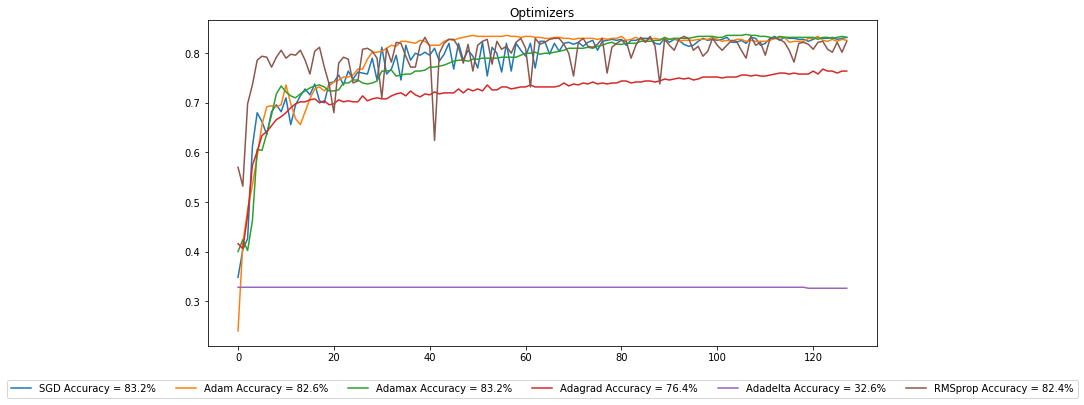Running the example creates a figure with 6 line plots showing the classification accuracy on the train and test sets of models with optimizers.

The plots show that certain optimizers dont converge. Some result in a more volatile learning process with higher variance in the classification accuracy.

## What is the Learning Rate?¶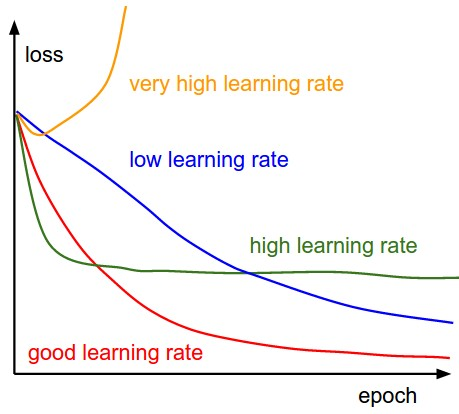The learning rate is a hyperparameter that controls how much to change the model in response to the estimated error each time the model weights are updated. Choosing the learning rate is challenging as a value too small may result in a long training process that could get stuck, whereas a value too large may result in learning a sub-optimal set of weights too fast or an unstable training process.

The learning rate may be the most important hyperparameter when configuring your neural network. Therefore it is vital to know how to investigate the effects of the learning rate on model performance and to build an intuition about the dynamics of the learning rate on model behavior.

In :
# fit a model and plot learning curve
def fit_model_lr(train_X, train_y, test_X, test_y, n_batch, epochs, opt, lr=None):

# define model
model = Sequential()

# compile model
model.compile(loss='categorical_crossentropy', optimizer=opt, metrics=['accuracy'])

# fit model
history = model.fit(train_X, train_y, validation_data=(test_X, test_y), epochs=epochs, verbose=0,
batch_size=n_batch)

# evaluate the model
scores = model.evaluate(test_X, test_y)
accuracy = round(scores * 100, 3)

# plot learning curves
plt.plot(history.history['accuracy'], label='train')
plt.plot(history.history['val_accuracy'], label='test')
plt.title(f'Accuracy = {accuracy}%, Learning Rate = {lr}', pad= -40)

plt.legend()

In :
plt.figure(figsize=(12,10))

rates = [1.0, 0.1, 0.01, 0.001, 0.0001, 0.00001, 0.000001, 0.0000001]

for i in range(len(rates)):

# determine the plot number
plot_no = 420 + (i+1)
plt.subplot(plot_no)

# fit model and plot learning curves
fit_model_lr(train_X, train_y, test_X, test_y, 256, epochs=128, opt=Adam(lr=rates[i]), lr=rates[i])

# show learning curves
plt.tight_layout()
plt.show()

500/500 [==============================] - 0s 11us/step
500/500 [==============================] - 0s 11us/step
500/500 [==============================] - 0s 13us/step
500/500 [==============================] - 0s 10us/step
500/500 [==============================] - 0s 10us/step
500/500 [==============================] - 0s 11us/step
500/500 [==============================] - 0s 10us/step
500/500 [==============================] - 0s 10us/step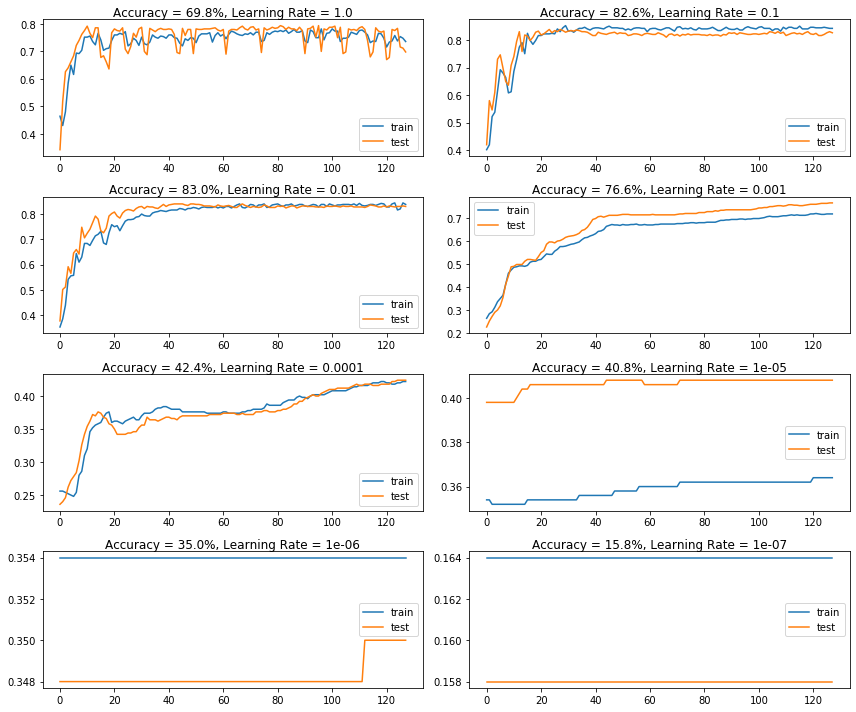Running the example creates a single figure that contains eight line plots for the eight different evaluated learning rates. Classification accuracy on the training dataset is marked in blue, whereas accuracy on the test dataset is marked in orange.

The plots show oscillations in behavior for the too-large learning rate of $1.0$ and the inability of the model to learn anything with the too-small learning rates of $1e^{-6}$ and $1e^{-7}$.

We can see that the model was able to learn the problem well with the learning rates $1e^{-1}$, $1e^{-2}$ and $1e^{-3}$, although successively slower as the learning rate was decreased. With the chosen model configuration, the results suggest a moderate learning rate of $0.01$ results in good model performance on the train and test sets.

## Momentum Dynamics¶

Momentum can smooth the progression of the learning algorithm that, in turn, can accelerate the training process.

We can adapt the example from the previous section to evaluate the effect of momentum with a fixed learning rate. In this case, we will choose the learning rate of $0.01$ that in the previous section converged to a reasonable solution.

The fit_model() function can be updated to take a “momentum” argument instead of a learning rate argument, that can be used in the configuration of the SGD class and reported on the resulting plot.

The updated version of this function is listed below.

In :
# fit a model and plot learning curve
def fit_model_mom(train_X, train_y, test_X, test_y, n_batch, epochs, opt, mom=None):

# define model
model = Sequential()

# compile model
model.compile(loss='categorical_crossentropy', optimizer=opt, metrics=['accuracy'])

# fit model
history = model.fit(train_X, train_y, validation_data=(test_X, test_y), epochs=epochs, verbose=0,
batch_size=n_batch)

# evaluate the model
scores = model.evaluate(test_X, test_y)
accuracy = round(scores * 100, 3)

# plot learning curves
plt.plot(history.history['accuracy'], label='train')
plt.plot(history.history['val_accuracy'], label='test')
plt.title(f'Accuracy = {accuracy}%, , momentum = {mom}', pad= -40)

plt.legend()

In :
plt.figure(figsize=(12,5))

mom = [0.0, 0.5, 0.9, 0.99]

for i in range(len(mom)):

# determine the plot number
plot_no = 220 + (i+1)
plt.subplot(plot_no)

# fit model and plot learning curves
fit_model_mom(train_X, train_y, test_X, test_y, 256, epochs=128, opt=SGD(lr=0.08, momentum=mom[i]), mom=mom[i])

# show learning curves
plt.tight_layout()
plt.show()

500/500 [==============================] - 0s 11us/step
500/500 [==============================] - 0s 11us/step
500/500 [==============================] - 0s 12us/step
500/500 [==============================] - 0s 10us/step